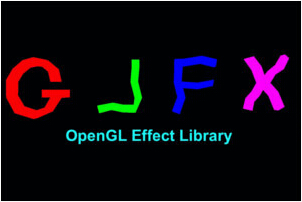modern opengl tutorial

end

# 第三十四课 GLFX —— 一个 OpenGL 效果库

## 代码

`` #include <glfx.h> ``

``int effect = glfxGenEffect(); ``

``````if (!glfxParseEffectFromFile(effect, "effect.glsl")) {
#ifdef __cplusplus // C++ error handling
std::string log = glfxGetEffectLog(effect);
std::cout << "Error parsing effect: " << log << std::endl;
#else // C error handling
char log;
glfxGetEffectLog(effect, log, sizeof(log));
printf("Error parsing effect: %s:\n", log);
#endif
return;
} ``````

``````int shaderProg = glfxCompileProgram(effect, "ProgramName");
// same error handling as above
} ``````

``glUseProgram(shaderProg); ``

``glfxDeleteEffect(effect); ``

``````program Lighting
{
vs(410)=VSmain();
fs(410)=FSmain();
}; ``````

``````void calculate_something()
{
...
}
{
calculate_something();
} ``````

``````struct VSoutput
{
vec2 TexCoord;
vec3 Normal;
};
shader VSmain(in vec3 Pos, in vec2 TexCoord, in vec3 Normal, out VSOutput VSout)
{
// do some transformations and update 'VSout'
VSout.TexCoord = TexCoord;
VSout.Normal = Normal;
}
shader FSmain(in VSOutput FSin, out vec4 FragColor)
{
// 'FSin' matches 'VSout' from the VS. Use it
// to do lighting calculations and write the final output to 'FragColor'
} ``````

‘include’关键字可用于包含其他的特效文件：

`` #include "another_effect.glsl" ``

``````struct VSInput2
{
vec3 Normal;
vec3 Tangent;
};
shader VSmain(in vec3 Pos : 5, in vec2 TexCoord : 6, in float colorScale : 10)``````

GLSL 提供了一些如 'flat' 和 'noperspective' 的修饰符，这些修饰符可用在从 VS 传递到 FS 的属性变量的前面。这些修饰符不能用在结构体成员变量的前面， GLFX 提供的一个解决方法是使用一个新的关键字 'interface' 来完成 'struct' 做不到的事。用 'interface' 定义的变量只能在不同着色器阶段之间执行，如果你需要将它全部传递到另一个函数中，那你需要先将其内容拷贝到结构体中，例如：

``````interface foo
{
flat int a;
noperspective float b;
};
struct bar
{
int a;
float b;
}
{
// ...
}
void Calc(bar c)
{
// ...
}
{
struct bar c;
c.a = f.a;
c.b = f.b;
Calc(c);
}``````

Tip：使用 'glfxc' 来验证特效文件

'glfxc' 是一个 GLFX 中的一个工具，它能解析特效文件，并使用本地 OpenGL 进行编译并报告发现的错误。可以通过如下方式运行它：

``glfxc <effect file name> <program name> ``

## 操作结果